# Selina Solutions Concise Mathematics Class 6 Chapter 9 Playing With Numbers Exercise 9(C)

Selina Solutions Concise Mathematics Class 6 Chapter 9 Playing With Numbers Exercise 9(C) provides basic fundamental concepts on the divisibility of numbers covered under this exercise. Students gain better conceptual knowledge by practising these solutions effectively. They can solve difficult questions by referring to solutions PDF and hence, also boost their confidence. Those who desire to score well in exams need to practice Selina Solutions created by experts, as per the ICSE guidelines. Download the Selina Solutions Concise Mathematics Class 6 Chapter 9 Playing With Numbers Exercise 9(C) PDF, from the links provided below

## Selina Solutions Concise Mathematics Class 6 Chapter 9: Playing With Numbers Exercise 9(C) Download PDF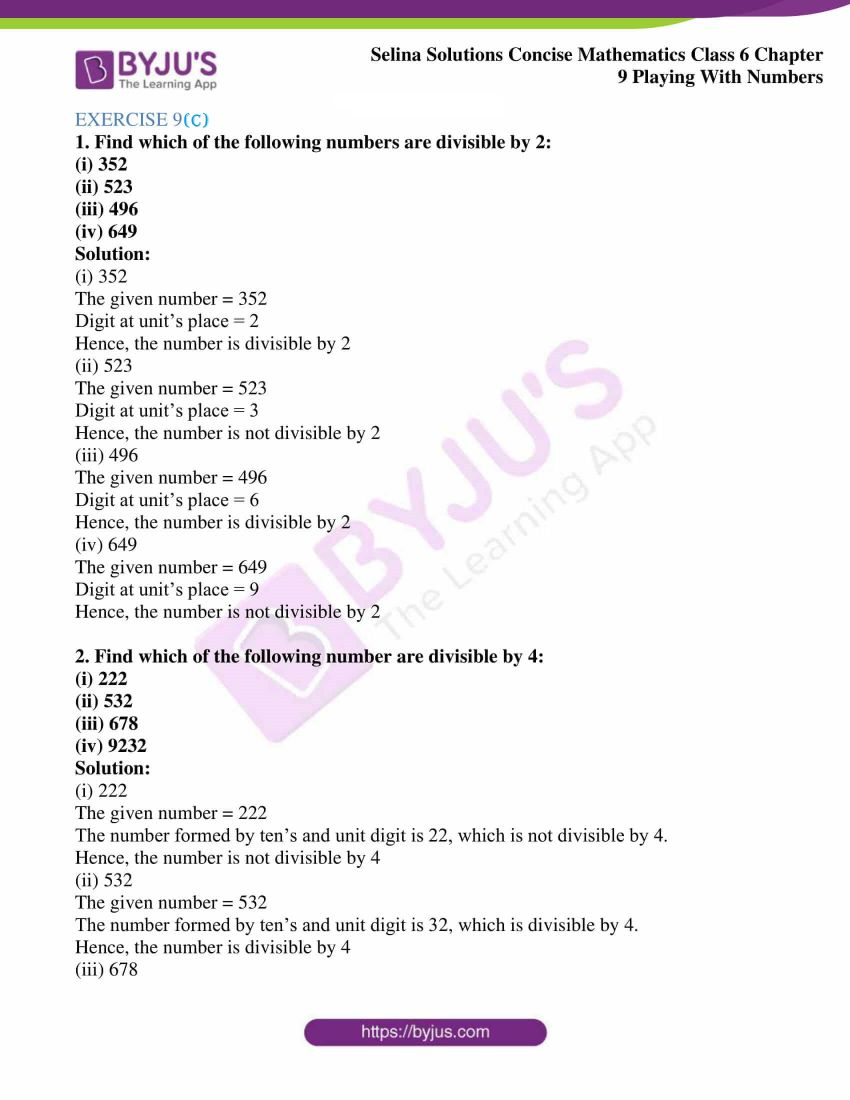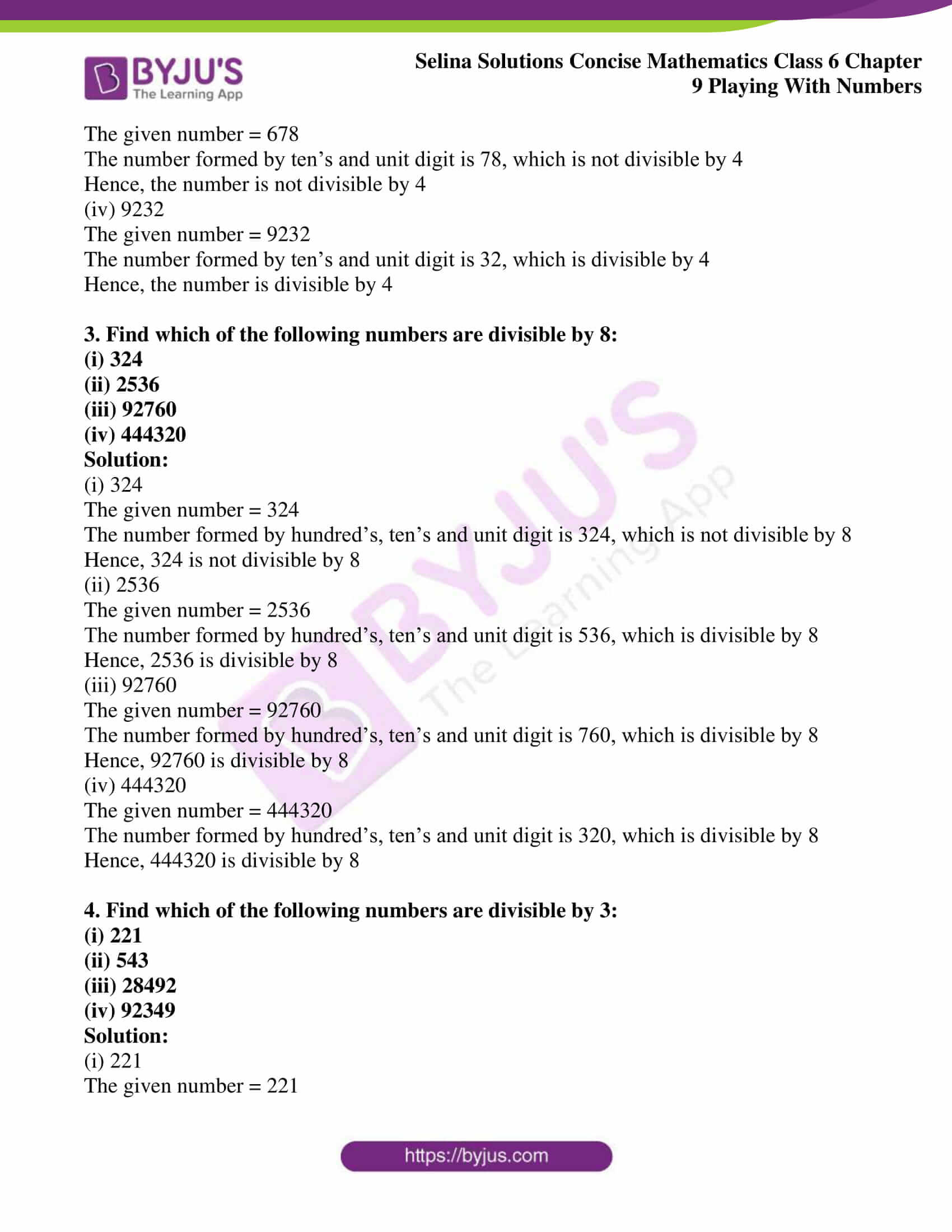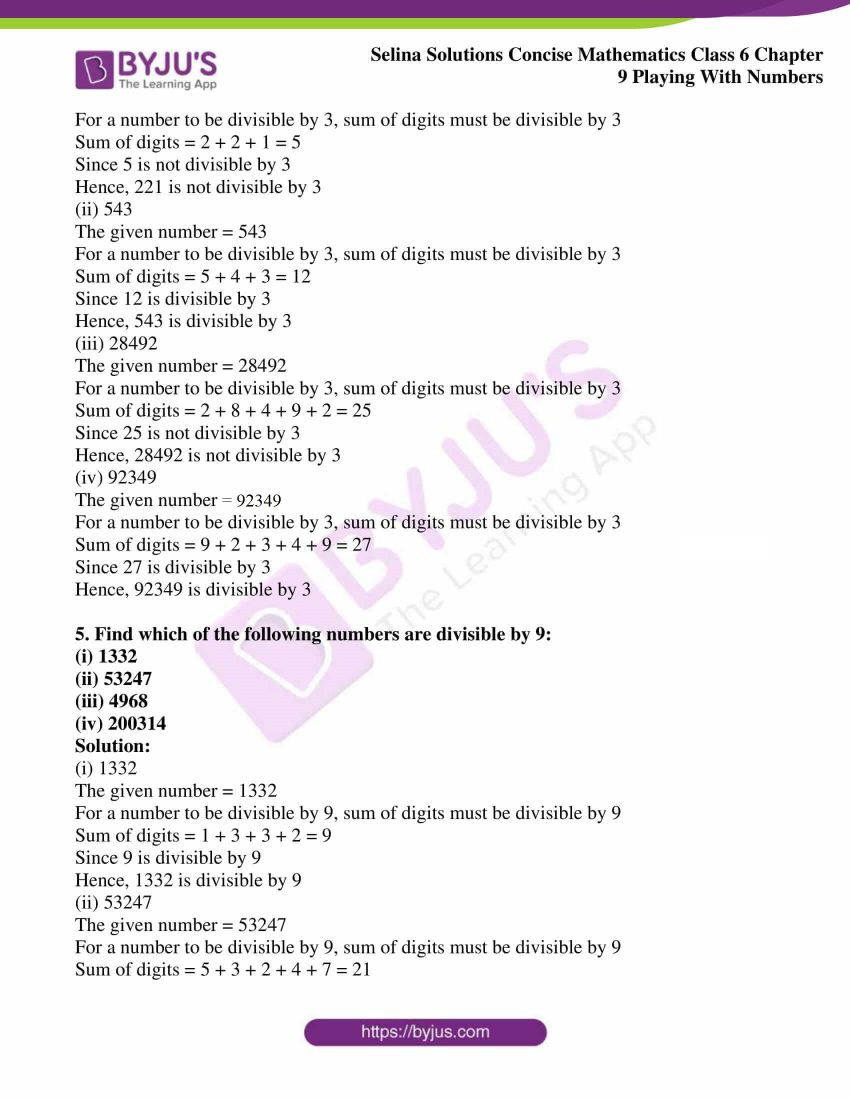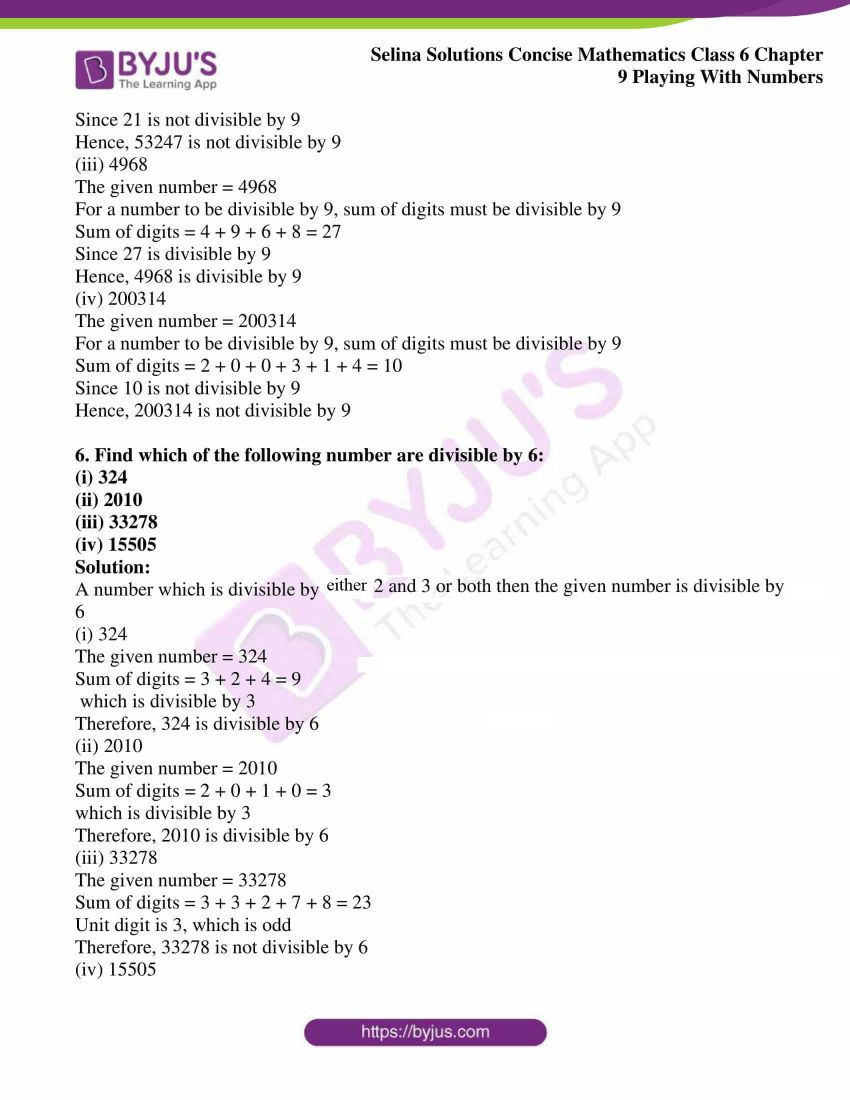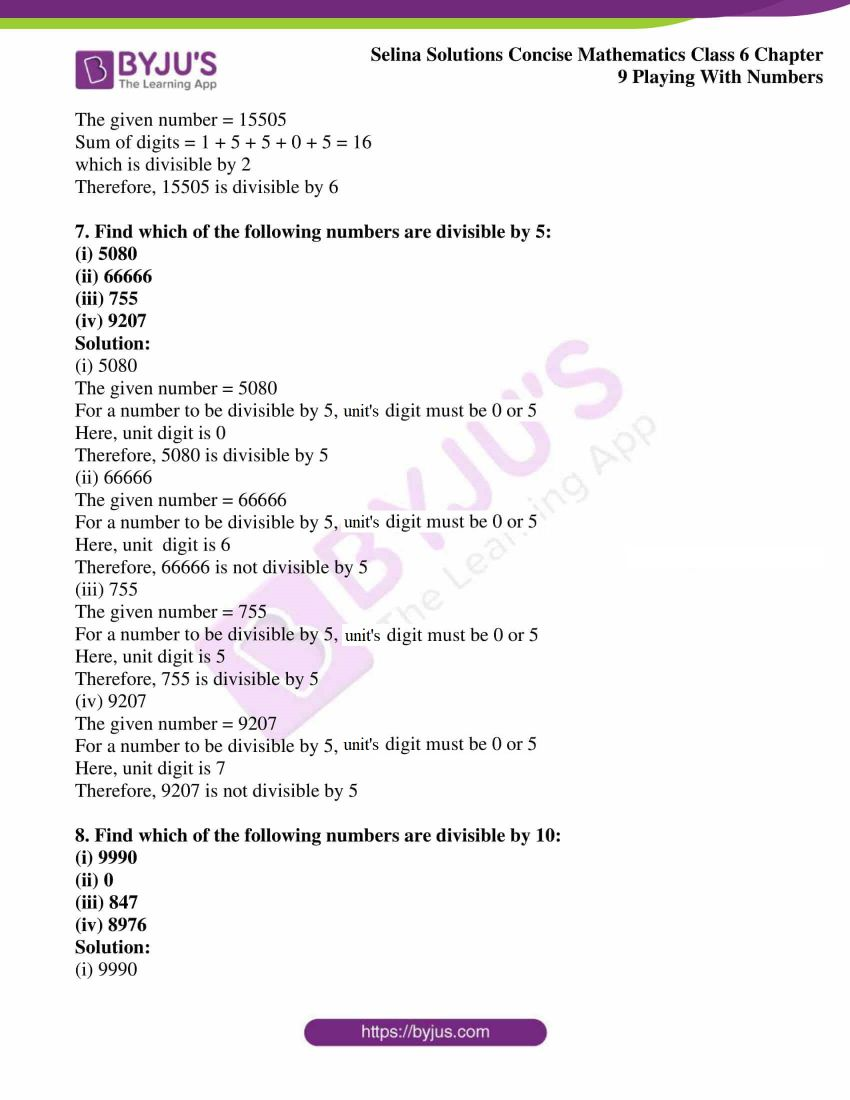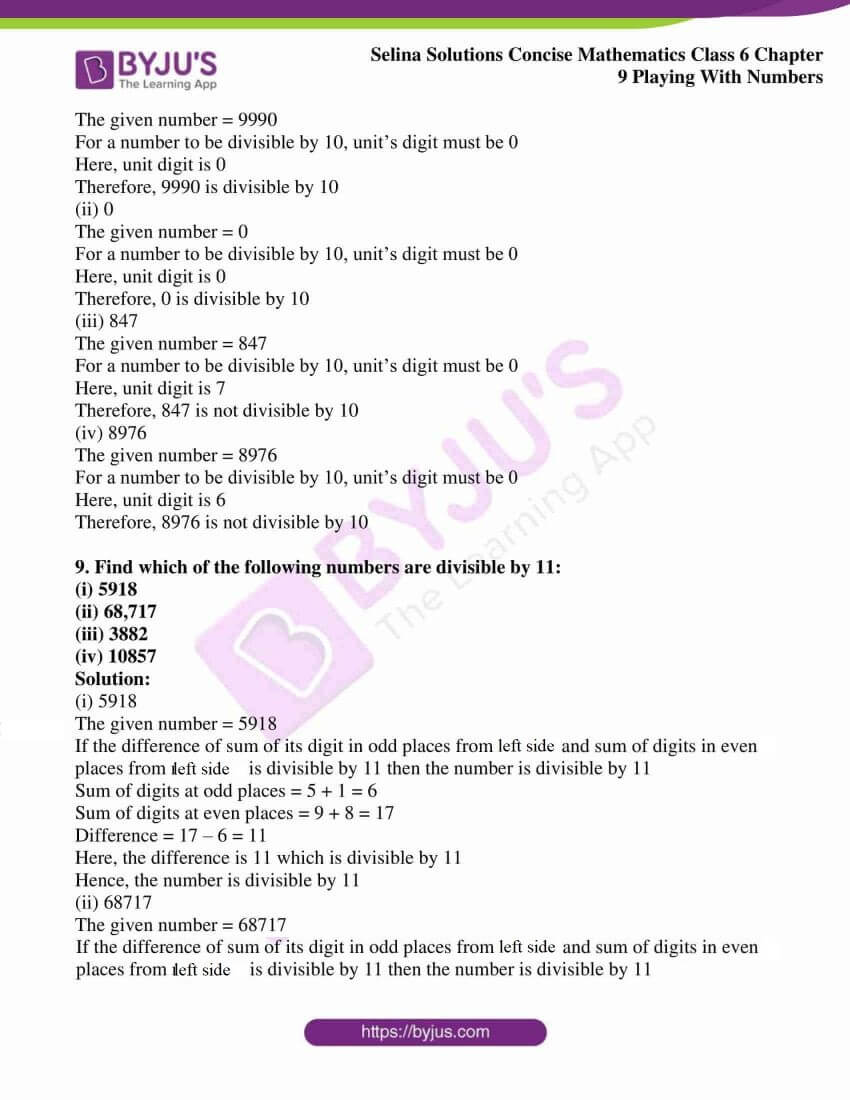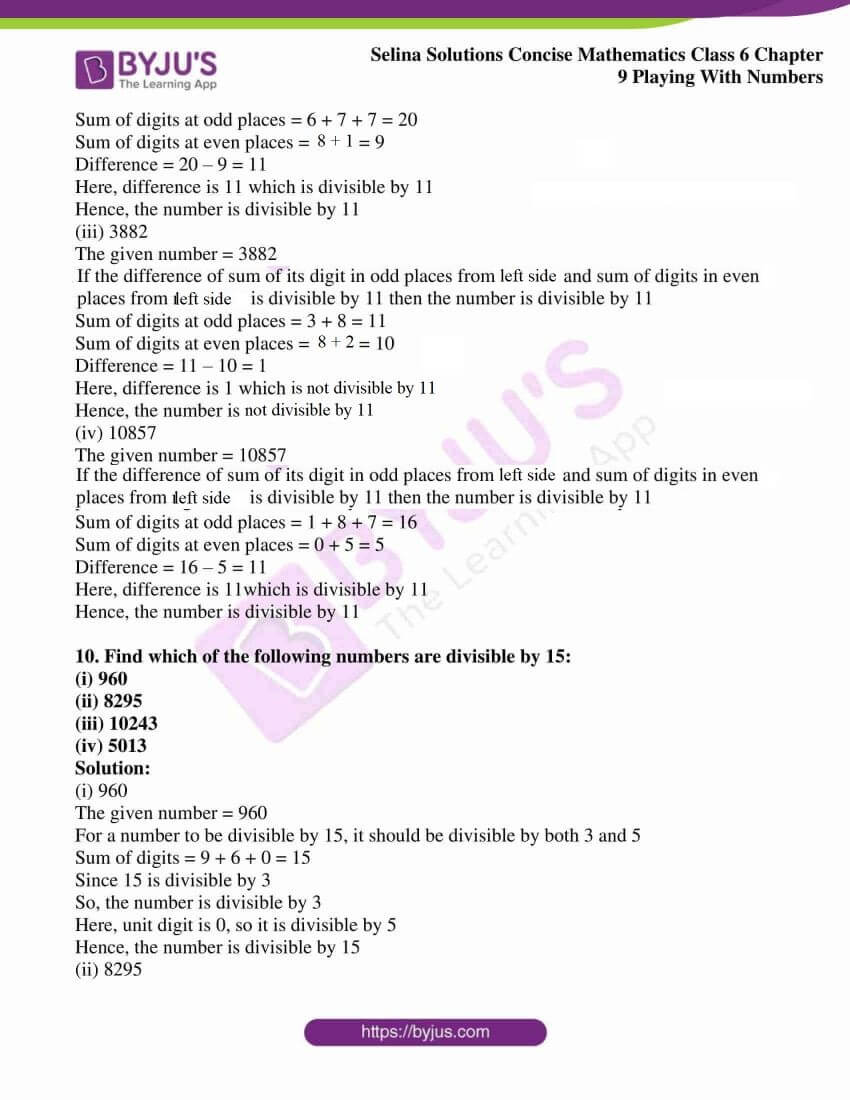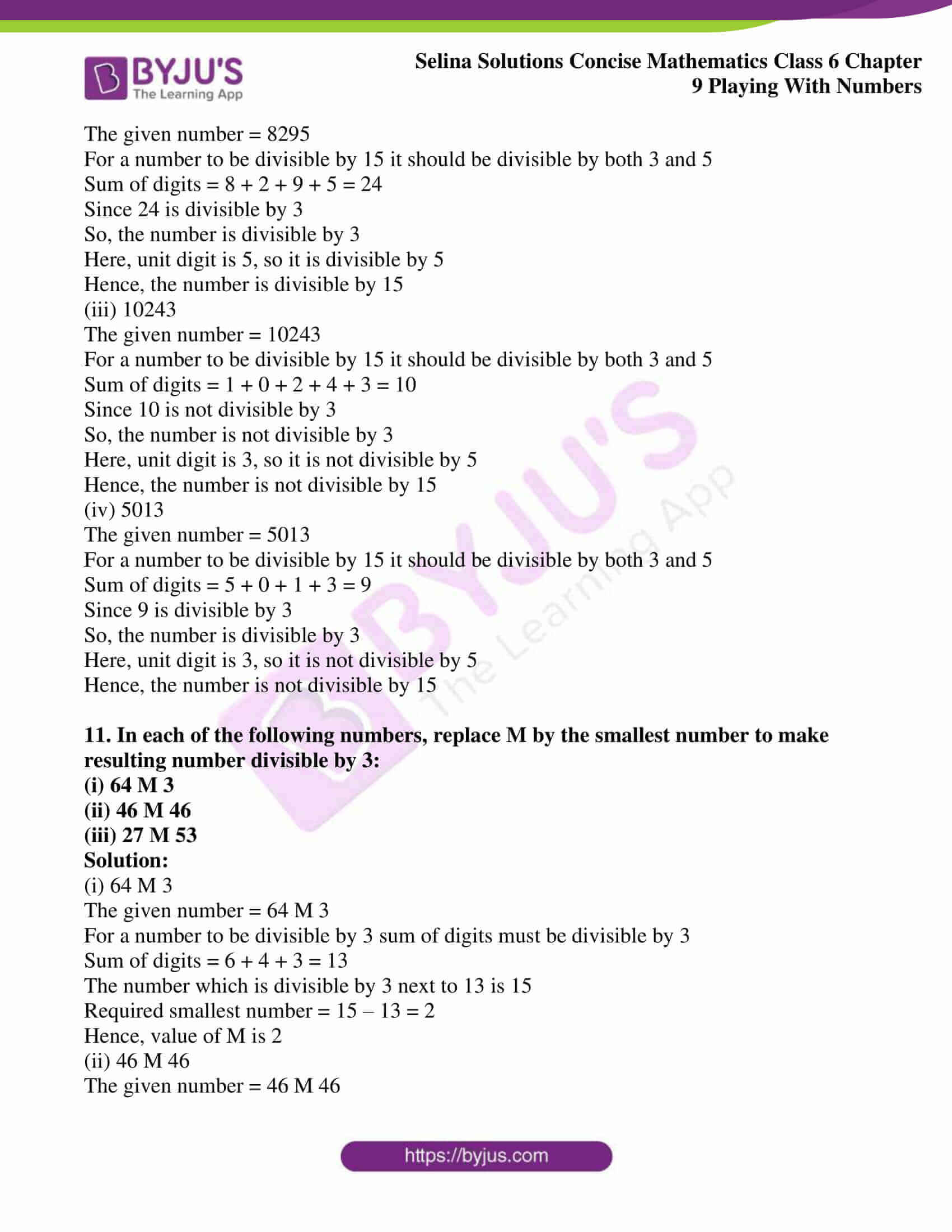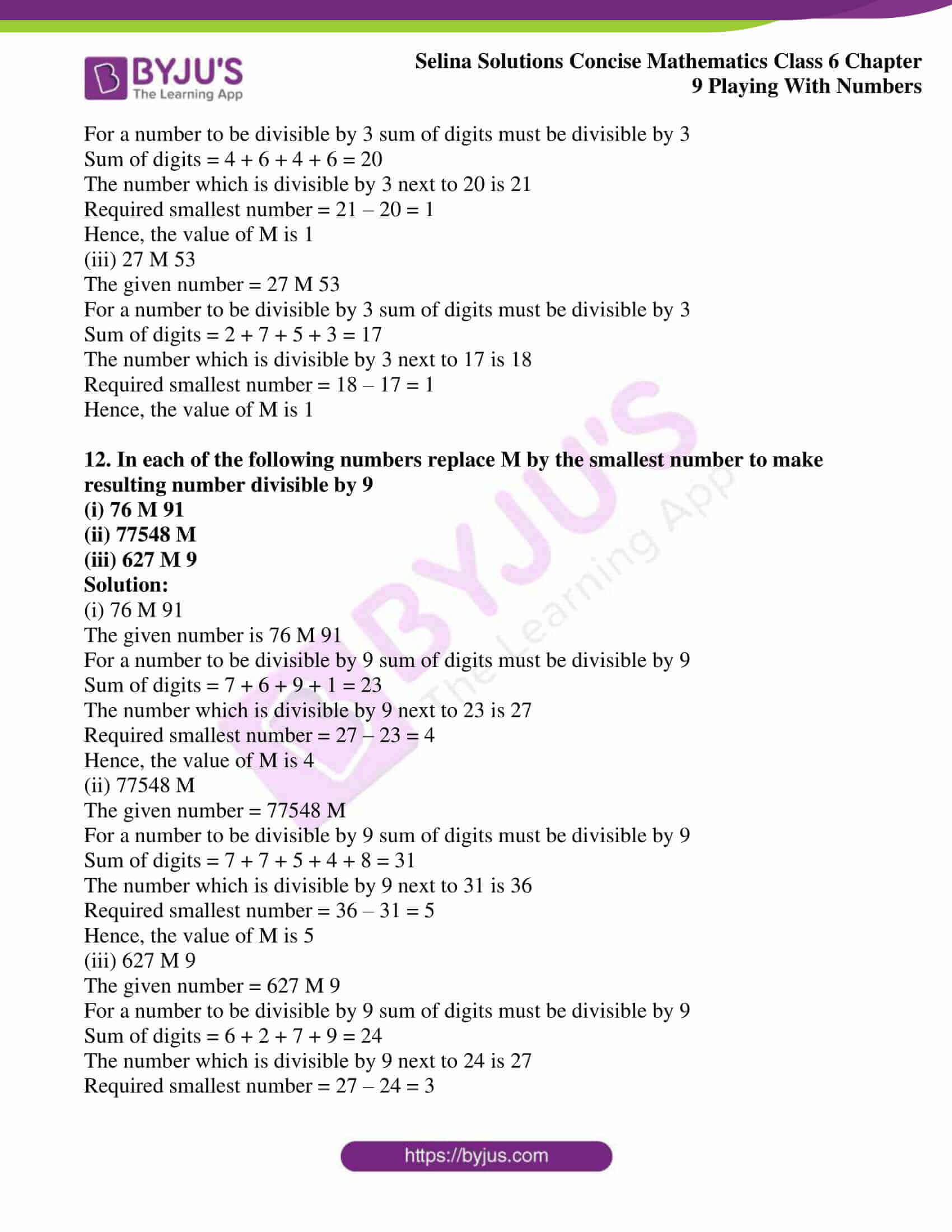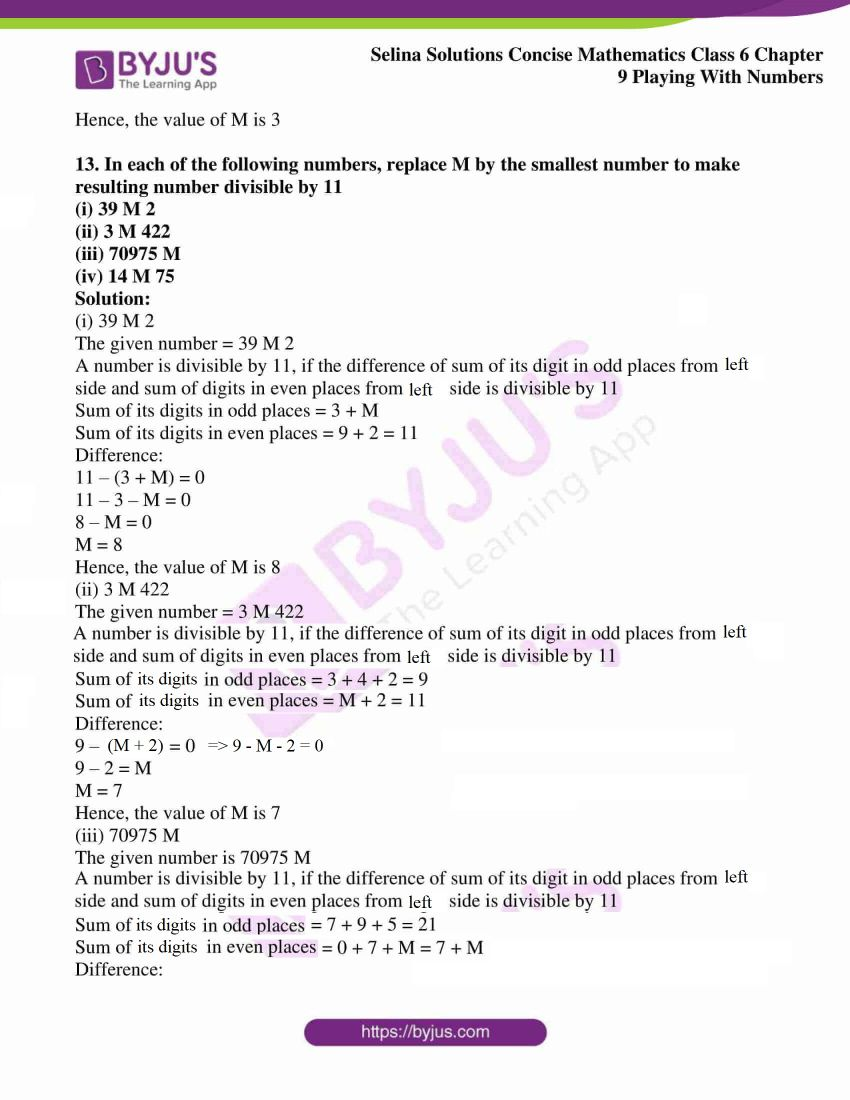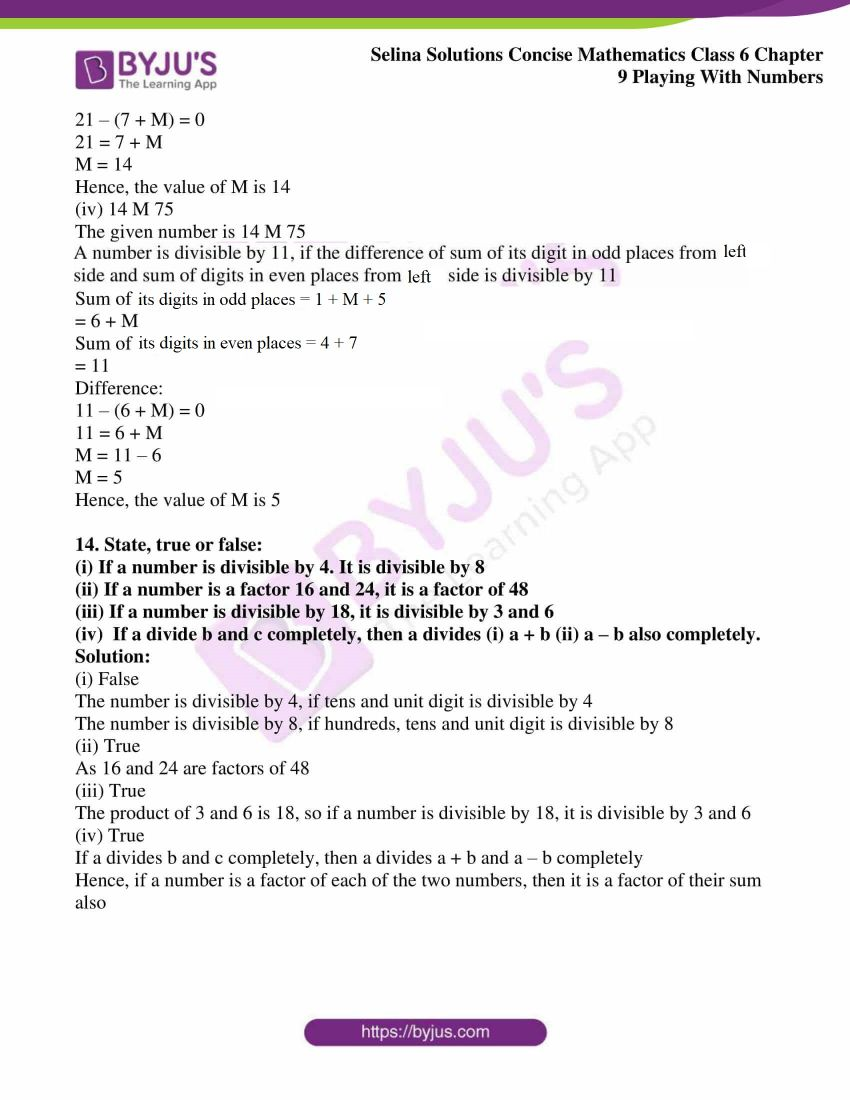### Access other exercises of Selina Solutions Concise Mathematics Class 6 Chapter 9 Playing With Numbers

Exercise 9(A) Solutions

Exercise 9(B) Solutions

### Access Selina Solutions Concise Mathematics Class 6 Chapter 9 Playing With Numbers Exercise 9(C)

#### Exercise 9(C)

1. Find which of the following numbers are divisible by 2:

(i) 352

(ii) 523

(iii) 496

(iv) 649

Solution:

(i) 352

The given number = 352

Digit at unit’s place = 2

Hence, the number is divisible by 2

(ii) 523

The given number = 523

Digit at unit’s place = 3

Hence, the number is not divisible by 2

(iii) 496

The given number = 496

Digit at unit’s place = 6

Hence, the number is divisible by 2

(iv) 649

The given number = 649

Digit at unit’s place = 9

Hence, the number is not divisible by 2

2. Find which of the following number are divisible by 4:

(i) 222

(ii) 532

(iii) 678

(iv) 9232

Solution:

(i) 222

The given number = 222

The number formed by ten’s and unit digit is 22, which is not divisible by 4.

Hence, the number is not divisible by 4

(ii) 532

The given number = 532

The number formed by ten’s and unit digit is 32, which is divisible by 4.

Hence, the number is divisible by 4

(iii) 678

The given number = 678

The number formed by ten’s and unit digit is 78, which is not divisible by 4

Hence, the number is not divisible by 4

(iv) 9232

The given number = 9232

The number formed by ten’s and unit digit is 32, which is divisible by 4

Hence, the number is divisible by 4

3. Find which of the following numbers are divisible by 8:

(i) 324

(ii) 2536

(iii) 92760

(iv) 444320

Solution:

(i) 324

The given number = 324

The number formed by hundred’s, ten’s and unit digit is 324, which is not divisible by 8

Hence, 324 is not divisible by 8

(ii) 2536

The given number = 2536

The number formed by hundred’s, ten’s and unit digit is 536, which is divisible by 8

Hence, 2536 is divisible by 8

(iii) 92760

The given number = 92760

The number formed by hundred’s, ten’s and unit digit is 760, which is divisible by 8

Hence, 92760 is divisible by 8

(iv) 444320

The given number = 444320

The number formed by hundred’s, ten’s and unit digit is 320, which is divisible by 8

Hence, 444320 is divisible by 8

4. Find which of the following numbers are divisible by 3:

(i) 221

(ii) 543

(iii) 28492

(iv) 92349

Solution:

(i) 221

The given number = 221

For a number to be divisible by 3, sum of digits must be divisible by 3

Sum of digits = 2 + 2 + 1 = 5

Since 5 is not divisible by 3

Hence, 221 is not divisible by 3

(ii) 543

The given number = 543

For a number to be divisible by 3, sum of digits must be divisible by 3

Sum of digits = 5 + 4 + 3 = 12

Since 12 is divisible by 3

Hence, 543 is divisible by 3

(iii) 28492

The given number = 28492

For a number to be divisible by 3, sum of digits must be divisible by 3

Sum of digits = 2 + 8 + 4 + 9 + 2 = 25

Since 25 is not divisible by 3

Hence, 28492 is not divisible by 3

(iv) 92349

The given number = 92349

For a number to be divisible by 3, sum of digits must be divisible by 3

Sum of digits = 9 + 2 + 3 + 4 + 9 = 27

Since 27 is divisible by 3

Hence, 92349 is divisible by 3

5. Find which of the following numbers are divisible by 9:

(i) 1332

(ii) 53247

(iii) 4968

(iv) 200314

Solution:

(i) 1332

The given number = 1332

For a number to be divisible by 9, sum of digits must be divisible by 9

Sum of digits = 1 + 3 + 3 + 2 = 9

Since 9 is divisible by 9

Hence, 1332 is divisible by 9

(ii) 53247

The given number = 53247

For a number to be divisible by 9, sum of digits must be divisible by 9

Sum of digits = 5 + 3 + 2 + 4 + 7 = 21

Since 21 is not divisible by 9

Hence, 53247 is not divisible by 9

(iii) 4968

The given number = 4968

For a number to be divisible by 9, sum of digits must be divisible by 9

Sum of digits = 4 + 9 + 6 + 8 = 27

Since 27 is divisible by 9

Hence, 4968 is divisible by 9

(iv) 200314

The given number = 200314

For a number to be divisible by 9, sum of digits must be divisible by 9

Sum of digits = 2 + 0 + 0 + 3 + 1 + 4 = 10

Since 10 is not divisible by 9

Hence, 200314 is not divisible by 9

6. Find which of the following number are divisible by 6:

(i) 324

(ii) 2010

(iii) 33278

(iv) 15505

Solution:

A number which is divisible by either 2 and 3 or both then the given number is divisible by 6

(i) 324

The given number = 324

Sum of digits = 3 + 2 + 4 = 9

which is divisible by 3

Therefore, 324 is divisible by 6

(ii) 2010

The given number = 2010

Sum of digits = 2 + 0 + 1 + 0 = 3

which is divisible by 3

Therefore, 2010 is divisible by 6

(iii) 33278

The given number = 33278

Sum of digits = 3 + 3 + 2 + 7 + 8 = 23

Unit digit is 3, which is odd

Therefore, 33278 is not divisible by 6

(iv) 15505

The given number = 15505

Sum of digits = 1 + 5 + 5 + 0 + 5 = 16

which is divisible by 2

Therefore, 15505 is divisible by 6

7. Find which of the following numbers are divisible by 5:

(i) 5080

(ii) 66666

(iii) 755

(iv) 9207

Solution:

(i) 5080

The given number = 5080

For a number to be divisible by 5, units digit must be 0 or 5

Here, unit digit is 0

Therefore, 5080 is divisible by 5

(ii) 66666

The given number = 66666

For a number to be divisible by 5, units digit must be 0 or 5

Here, unit digit is 6

Therefore, 66666 is not divisible by 5

(iii) 755

The given number = 755

For a number to be divisible by 5, units digit must be 0 or 5

Here, unit digit is 5

Therefore, 755 is divisible by 5

(iv) 9207

The given number = 9207

For a number to be divisible by 5, units digit must be 0 or 5

Here, unit digit is 7

Therefore, 9207 is not divisible by 5

8. Find which of the following numbers are divisible by 10:

(i) 9990

(ii) 0

(iii) 847

(iv) 8976

Solution:

(i) 9990

The given number = 9990

For a number to be divisible by 10, unit’s digit must be 0

Here, unit digit is 0

Therefore, 9990 is divisible by 10

(ii) 0

The given number = 0

For a number to be divisible by 10, unit’s digit must be 0

Here, unit digit is 0

Therefore, 0 is divisible by 10

(iii) 847

The given number = 847

For a number to be divisible by 10, unit’s digit must be 0

Here, unit digit is 7

Therefore, 847 is not divisible by 10

(iv) 8976

The given number = 8976

For a number to be divisible by 10, unit’s digit must be 0

Here, unit digit is 6

Therefore, 8976 is not divisible by 10

9. Find which of the following numbers are divisible by 11:

(i) 5918

(ii) 68,717

(iii) 3882

(iv) 10857

Solution:

(i) 5918

The given number = 5918

If the difference of sum of its digit in odd places from left side and sum of digits in even places from left side is divisible by 11 then the number is divisible by 11

Sum of digits at odd places = 5 + 1 = 6

Sum of digits at even places = 9 + 8 = 17

Difference = 17 – 6 = 11

Here, the difference is 11 which is divisible by 11

Hence, the number is divisible by 11

(ii) 68717

The given number = 68717

If the difference of sum of its digit in odd places from left side and sum of digits in even places from left side is divisible by 11 then the number is divisible by 11

Sum of digits at odd places = 6 + 7 + 7 = 20

Sum of digits at even places = 8 + 1 = 9

Difference = 20 – 9 = 11

Here, difference is 11 which is divisible by 11

Hence, the number is divisible by 11

(iii) 3882

The given number = 3882

If the difference of sum of its digit in odd places from left side and sum of digits in even places from left side is divisible by 11 then the number is divisible by 11

Sum of digits at odd places = 3 + 8 = 11

Sum of digits at even places = 8 + 2 = 10

Difference = 11 – 10 = 1

Here, difference is 1 which is not divisible by 11

Hence, the number is not divisible by 11

(iv) 10857

The given number = 10857

If the difference of sum of its digit in odd places from left side and sum of digits in even places from left side is divisible by 11 then the number is divisible by 11

Sum of digits at odd places = 1 + 8 + 7 = 16

Sum of digits at even places = 0 + 5 = 5

Difference = 16 – 5 = 11

Here, difference is 11which is divisible by 11

Hence, the number is divisible by 11

10. Find which of the following numbers are divisible by 15:

(i) 960

(ii) 8295

(iii) 10243

(iv) 5013

Solution:

(i) 960

The given number = 960

For a number to be divisible by 15, it should be divisible by both 3 and 5

Sum of digits = 9 + 6 + 0 = 15

Since 15 is divisible by 3

So, the number is divisible by 3

Here, unit digit is 0, so it is divisible by 5

Hence, the number is divisible by 15

(ii) 8295

The given number = 8295

For a number to be divisible by 15 it should be divisible by both 3 and 5

Sum of digits = 8 + 2 + 9 + 5 = 24

Since 24 is divisible by 3

So, the number is divisible by 3

Here, unit digit is 5, so it is divisible by 5

Hence, the number is divisible by 15

(iii) 10243

The given number = 10243

For a number to be divisible by 15 it should be divisible by both 3 and 5

Sum of digits = 1 + 0 + 2 + 4 + 3 = 10

Since 10 is not divisible by 3

So, the number is not divisible by 3

Here, unit digit is 3, so it is not divisible by 5

Hence, the number is not divisible by 15

(iv) 5013

The given number = 5013

For a number to be divisible by 15 it should be divisible by both 3 and 5

Sum of digits = 5 + 0 + 1 + 3 = 9

Since 9 is divisible by 3

So, the number is divisible by 3

Here, unit digit is 3, so it is not divisible by 5

Hence, the number is not divisible by 15

11. In each of the following numbers, replace M by the smallest number to make resulting number divisible by 3:

(i) 64 M 3

(ii) 46 M 46

(iii) 27 M 53

Solution:

(i) 64 M 3

The given number = 64 M 3

For a number to be divisible by 3 sum of digits must be divisible by 3

Sum of digits = 6 + 4 + 3 = 13

The number which is divisible by 3 next to 13 is 15

Required smallest number = 15 – 13 = 2

Hence, value of M is 2

(ii) 46 M 46

The given number = 46 M 46

For a number to be divisible by 3 sum of digits must be divisible by 3

Sum of digits = 4 + 6 + 4 + 6 = 20

The number which is divisible by 3 next to 20 is 21

Required smallest number = 21 – 20 = 1

Hence, the value of M is 1

(iii) 27 M 53

The given number = 27 M 53

For a number to be divisible by 3 sum of digits must be divisible by 3

Sum of digits = 2 + 7 + 5 + 3 = 17

The number which is divisible by 3 next to 17 is 18

Required smallest number = 18 – 17 = 1

Hence, the value of M is 1

12. In each of the following numbers replace M by the smallest number to make resulting number divisible by 9

(i) 76 M 91

(ii) 77548 M

(iii) 627 M 9

Solution:

(i) 76 M 91

The given number is 76 M 91

For a number to be divisible by 9 sum of digits must be divisible by 9

Sum of digits = 7 + 6 + 9 + 1 = 23

The number which is divisible by 9 next to 23 is 27

Required smallest number = 27 – 23 = 4

Hence, the value of M is 4

(ii) 77548 M

The given number = 77548 M

For a number to be divisible by 9 sum of digits must be divisible by 9

Sum of digits = 7 + 7 + 5 + 4 + 8 = 31

The number which is divisible by 9 next to 31 is 36

Required smallest number = 36 – 31 = 5

Hence, the value of M is 5

(iii) 627 M 9

The given number = 627 M 9

For a number to be divisible by 9 sum of digits must be divisible by 9

Sum of digits = 6 + 2 + 7 + 9 = 24

The number which is divisible by 9 next to 24 is 27

Required smallest number = 27 – 24 = 3

Hence, the value of M is 3

13. In each of the following numbers, replace M by the smallest number to make resulting number divisible by 11

(i) 39 M 2

(ii) 3 M 422

(iii) 70975 M

(iv) 14 M 75

Solution:

(i) 39 M 2

The given number = 39 M 2

A number is divisible by 11, if the difference of sum of its digit in odd places from left side and sum of digits in even places from left side is divisible by 11

Sum of its digits in odd places = 3 + M

Sum of its digits in even places = 9 + 2 = 11

Difference:

11 – (3 + M) = 0

11 – 3 – M = 0

8 – M = 0

M = 8

Hence, the value of M is 8

(ii) 3 M 422

The given number = 3 M 422

A number is divisible by 11, if the difference of sum of its digit in odd places from left side and sum of digits in even places from left side is divisible by 11

Sum of its digits in odd places = 3 + 4 + 2 = 9

Sum of its digits in even places = M + 2 = 11

Difference:

9 – (M + 2) = 0

9 –  M – 2 = 0

9 – M = 2

M = 7

Hence, the value of M is 7

(iii) 70975 M

The given number is 70975 M

A number is divisible by 11, if the difference of sum of its digit in odd places from left side and sum of digits in even places from left side is divisible by 11

Sum of its digits in odd places = 7 + 9 + 5 = 21

Sum of its digits in even places = 0 + 7 + M = 7 + M

Difference:

21 – (7 + M) = 0

21 = 7 + M

M = 14

Hence, the value of M is 14

(iv) 14 M 75

The given number is 14 M 75

A number is divisible by 11, if the difference of sum of its digit in odd places from left side and sum of digits in even places from left side is divisible by 11

Sum of its digits in odd places = 1 + M + 5

= 6 + M

Sum of its digits in even places = 4 + 7

= 11

Difference:

11 – (6 + M) = 0

11 = 6 + M

M = 11 – 6

M = 5

Hence, the value of M is 5

14. State, true or false:

(i) If a number is divisible by 4. It is divisible by 8

(ii) If a number is a factor 16 and 24, it is a factor of 48

(iii) If a number is divisible by 18, it is divisible by 3 and 6

(iv) If a divide b and c completely, then a divides (i) a + b (ii) a – b also completely.

Solution:

(i) False

The number is divisible by 4, if tens and unit digit is divisible by 4

The number is divisible by 8, if hundreds, tens and unit digit is divisible by 8

(ii) True

As 16 and 24 are factors of 48

(iii) True

The product of 3 and 6 is 18, so if a number is divisible by 18, it is divisible by 3 and 6

(iv) True

If a divides b and c completely, then a divides a + b and a – b completely

Hence, if a number is a factor of each of the two numbers, then it is a factor of their sum also